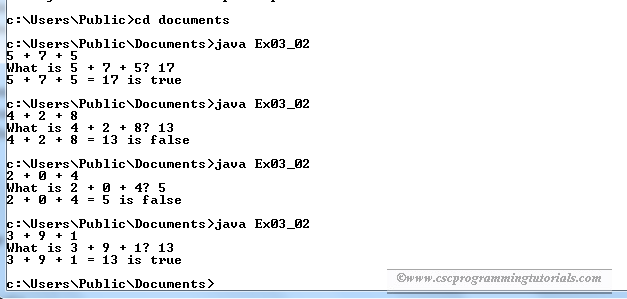# Exercise 3.2: Game: Add Three Numbers

The program below is the solution to Liang's Introduction to Java Programming (9th Edition) Chapter 3 Exercise 3.2.

Question: The program in Listing 3.1 generates two integers and prompts the user to enter the sum of these two integers. Revise the program to generate three single-digit integers and prompt the user to enter the sum of these three integers.
```/**
*
* @Author: Aghatise Osazuwa
* Website: www.cscprogrammingtutorials.com
*
* Exercise 3.2 - Game: add three numbers
*/

import java.util.Scanner;

public class Ex03_02 {
public static void main(String[] args) {
// declare variables
int number1 = (int)(System.currentTimeMillis() % 10);
int number2 = (int)(System.currentTimeMillis() / 7 % 10);
int number3 = (int)(System.currentTimeMillis() * 7 % 10);

// create Scanner to read user input
Scanner input = new Scanner(System.in);
System.out.print(number1 + " + " + number2 + " + " + number3);
// prompt user to enter details
System.out.print("\nWhat is " + number1 + " + " + number2 + " + " + number3 + "? ");
int answer = input.nextInt();
System.out.println(number1 + " + " + number2 + " + " + number3 + " = " +
answer + " is " + (number1 + number2 + number3 == answer));
}
}
```Click here to see other solutions to Introduction to Java Programming.